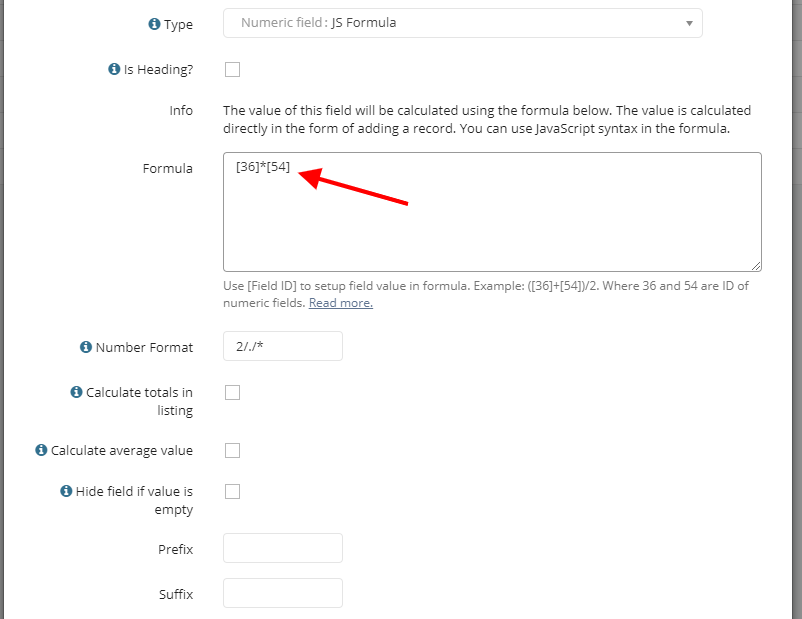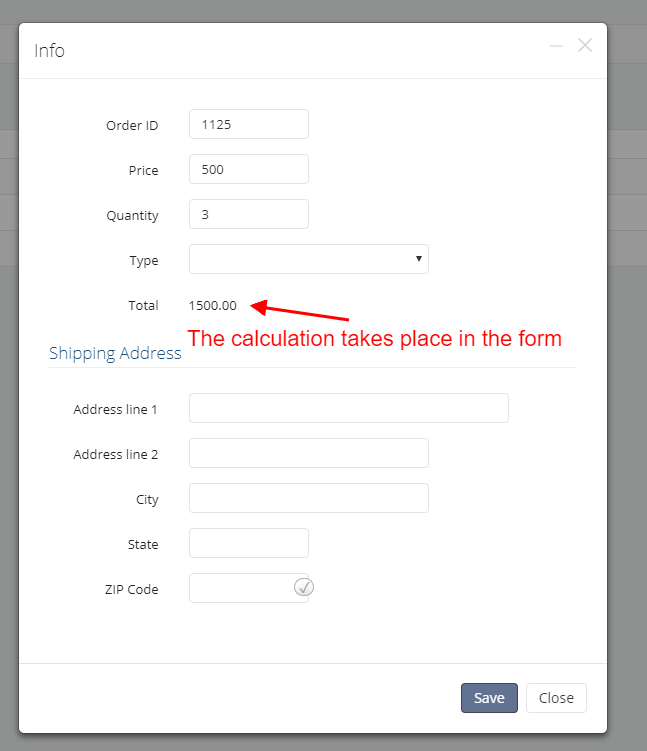Sometimes it becomes necessary to perform calculations directly in the form of a record when entering data. For example, calculate the amount depending on the quantity. To solve this task, a special type of field "JS Formula" has been developed.

In the field settings, in the "Formula" option, you must enter a mathematical formula, where you need to use the field ID as arguments. The values of these fields will be using during  the calculation.Mathematical calculations occur directly in the form of adding/editing a record when entering data. The calculations take place using JavaScript, which allows you to use any mathematical functions and operators of JavaScript language.Simple calculations

`(+)/2`

where 36 and 54 — id of numeric fields.

Calculation by the formula with the condition

`(>5) ? (+)/2:0`

Custom Function

If you need more logic, then you can use the following example:

```my_function();
function my_function()
{
my_value = *+get_value()
if( my_value>500)
{
return my_value*2
}
else
{
return my_value
}
}```